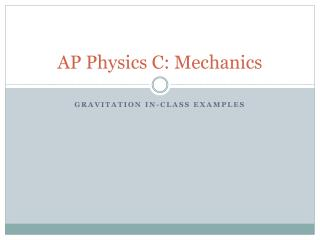DownloadDownload PresentationAP Physics C: Mechanics

# AP Physics C: Mechanics

Télécharger la présentation## AP Physics C: Mechanics

- - - - - - - - - - - - - - - - - - - - - - - - - - - E N D - - - - - - - - - - - - - - - - - - - - - - - - - - -
##### Presentation Transcript

1. AP Physics C: Mechanics Gravitation in-class examples

2. Example 1 – Gravitational Attraction Given that the radius of Earth is 6.37 x 106 m, determine the mass of Earth.

3. Example 1 – Gravitational Attraction Given that the radius of Earth is 6.37 x 106 m, determine the mass of Earth. 6.0 x 1024 kg

4. Example 2 – Gravitational Attraction Communications satellites are often parked in geosynchronous orbits above Earth’s surface. Such satellites have orbit periods that are equal to Earth’s rotation period, so they remain above the same position on Earth’s surface. Determine the altitude and the speed that a satellite must have to be in a geosynchronous orbit above a fixed point on Earth’s equator. (The mass of Earth is 5.98 x 1024 kg).

5. Example 2 – Gravitational Attraction Communications satellites are often parked in geosynchronous orbits above Earth’s surface. Such satellites have orbit periods that are equal to Earth’s rotation period, so they remain above the same position on Earth’s surface. Determine the altitude and the speed that a satellite must have to be in a geosynchronous orbit above a fixed point on Earth’s equator. (The mass of Earth is 5.98 x 1024 kg). 3070 m/s

6. Example 3 – Gravitational Attraction A uniform, slender bar of mass M has length L. Determine the gravitational force it exerts on the point particle of mass m shown below: m M |-----z--------|---------------------L--------------------|

7. Example 3 – Gravitational Attraction A uniform, slender bar of mass M has length L. Determine the gravitational force it exerts on the point particle of mass m shown below: m M |-----z--------|---------------------L--------------------|

8. Example 4 – Gravitational Potential With what minimum speed must an object of mass m be launched in order to escape Earth’s gravitational field? (This is called escape speed, vesc)

9. Example 4 – Gravitational Potential With what minimum speed must an object of mass m be launched in order to escape Earth’s gravitational field? (This is called escape speed, vesc) 1.12 x 104 m/s

10. Example 5 – Gravitational Potential A satellite of mass m is in a circular orbit of radius R around Earth (radius rE,mass M). What is its total mechanical energy (where Ugrav is considered zero as R approaches infinity)?

11. Example 5 – Gravitational Potential A satellite of mass m is in a circular orbit of radius R around Earth (radius rE,mass M). What is its total mechanical energy (where Ugrav is considered zero as R approaches infinity)?

12. Example 5 – Gravitational Potential A satellite of mass m is in a circular orbit of radius R around Earth (radius rE,mass M). How much work would be required to move the satellite into a new orbit, with radius 2R?

13. Example 5 – Gravitational Potential A satellite of mass m is in a circular orbit of radius R around Earth (radius rE,mass M). How much work would be required to move the satellite into a new orbit, with radius 2R?

14. Example 8– Kepler’s Laws An artificial satellite of mass m travels at a constant speed in a circular orbit of radius R around Earth (mass M). What is the speed of the satellite?

15. Example 6– Kepler’s Laws Assume that asteroid mass m is much less than Sun mass M. What is the total energy of the asteroid? What is the ratio of v1 (the asteroid’s speed at aphelion) to v2(the asteroid’s speed at perihelion?) in terms of r1and r2. What is the time necessary for the asteroid to make a complete orbit around the Sun?

16. Example 7– Kepler’s Laws For the barycentric diagram, derive a corrected version of Kepler’s Third Law for the orbiting system. Both bodies have orbit period T.